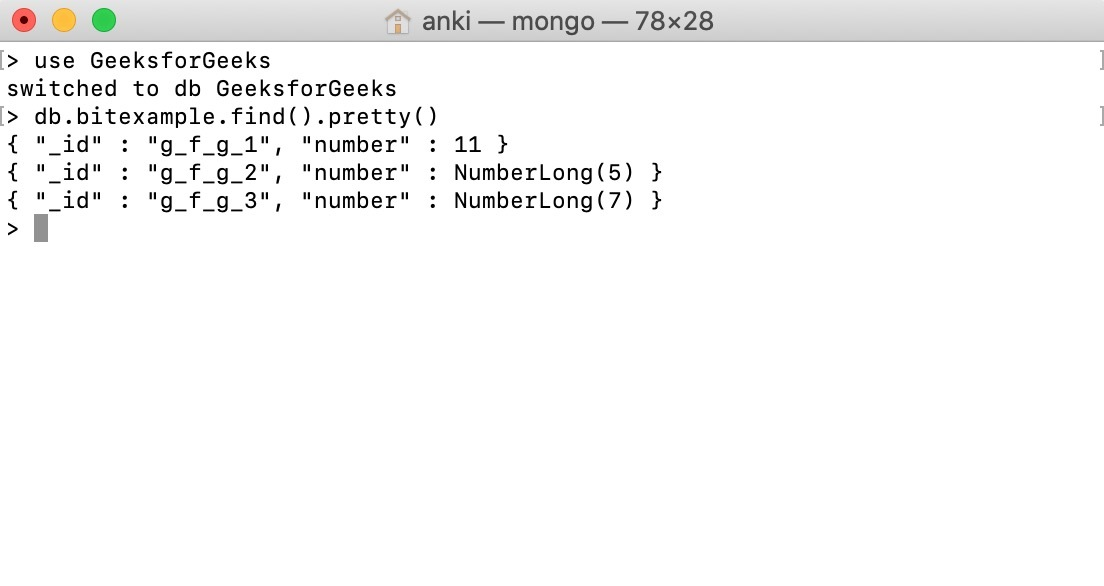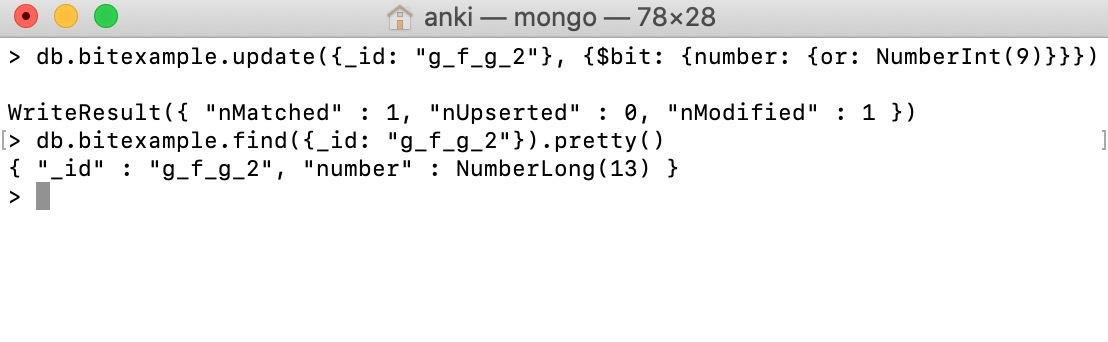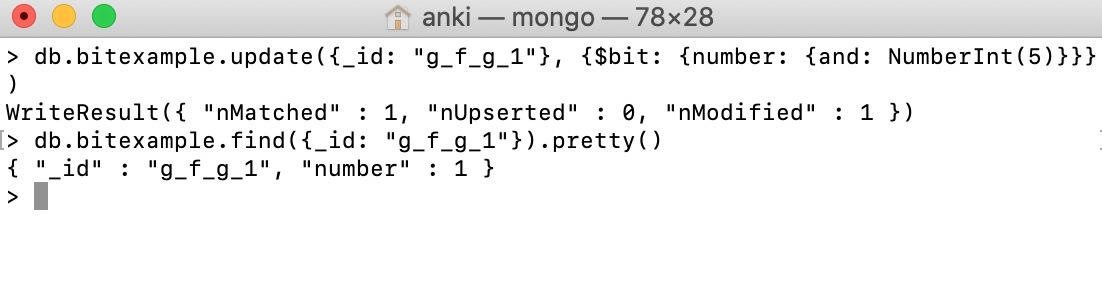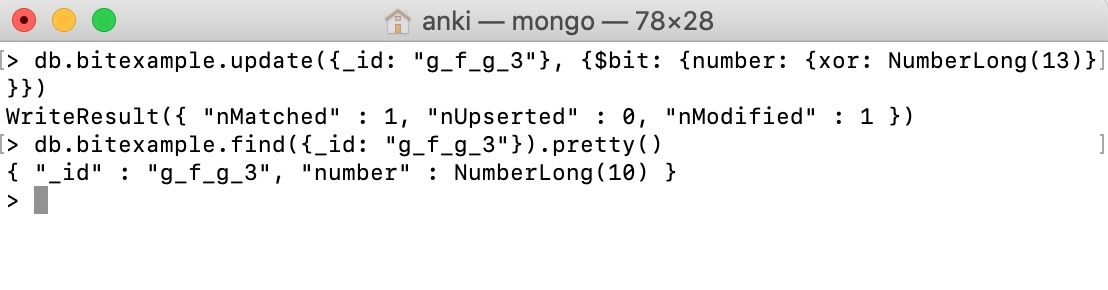# MongoDB Bitwise Update Operator

MongoDB provides a \$bit operator to perform a bitwise update of a field. This operator supports bitwise xor, bitwise or, and bitwise and operations.

Syntax:

```{ \$bit: { <field1>: { <or|and|xor>: <int> } } }
```

Important Points:

• Use \$bit operator only with integer fields(either 32-bit integer or 64-bit integer)
• To specify a field in embedded/nested documents or in an array use dot notation.
• All the numbers in the mongo shell are double not an integer. So, you need to use NumberInt() or the NumberLong() constructor to specify integers.
• You can use this operator in methods like update(), findAndModify(), etc., according to your requirements.

In the following examples, we are working with:

Database: GeeksforGeeks
Collection: bitexample
Document: three documents that contain the data in the form of field-value pairs.## Bitwise OR:

In this example, we are using update() method to update the value of number field of the document(id: g_f_g_2) to the result(i.e. NumberLong(13) or 1101) of a bitwise or operation perform between the current value NumberLong(5) (i.e. 0101) and NumberInt(9) (i.e. 1001):

```db.bitexample.update({_id: "g_f_g_2"},
{\$bit: {number: {or: NumberInt(9)}}})
```## Bitwise AND:

In this example, we are using update() method to update the value of number field of the document(id: g_f_g_1) to the result(i.e. NumberInt(1) or 0001) of a bitwise and operation perform between the current value NumberInt(11) (i.e. 1011) and NumberInt(5) (i.e. 0101):

```db.bitexample.update({_id: "g_f_g_1"},
{\$bit: {number: {and: NumberInt(5)}}})
```## Bitwise XOR:

In this example, we are using update() method to update the value of number field of the document(id: g_f_g_3) to the result(i.e. NumberLong(10) or 1010) of a bitwise xor operation perform between the current value NumberLong(7) (i.e. 0111) and NumberLong(13) (i.e. 1101):

```db.bitexample.update({_id: "g_f_g_3"},
{\$bit: {number: {xor: NumberLong(13)}}})
```My Personal Notes arrow_drop_upCheck out this Author's contributed articles.

If you like GeeksforGeeks and would like to contribute, you can also write an article using contribute.geeksforgeeks.org or mail your article to contribute@geeksforgeeks.org. See your article appearing on the GeeksforGeeks main page and help other Geeks.

Please Improve this article if you find anything incorrect by clicking on the "Improve Article" button below.

Article Tags :

Be the First to upvote.

Please write to us at contribute@geeksforgeeks.org to report any issue with the above content.